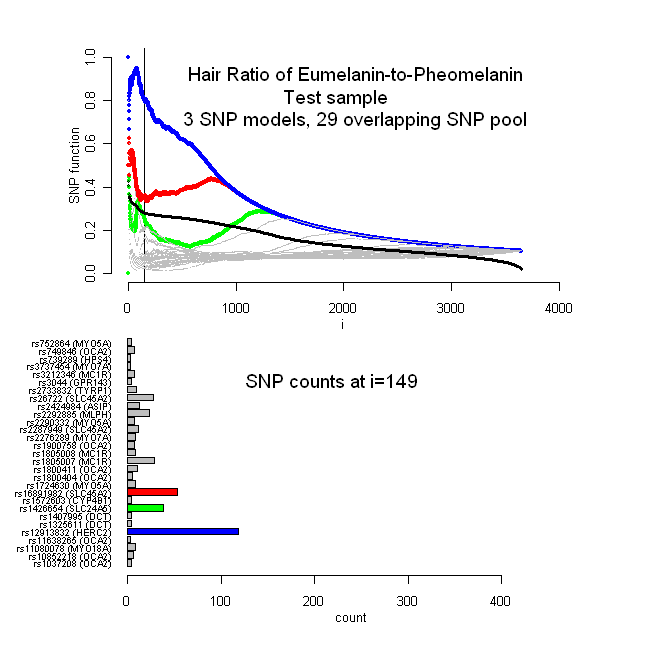Figure 8: (A) Three-SNP multiple linear regression (MLR) models for natural log of the hair ratios melanins across populations (test sample set). The horizontalaxis depicts all 3654 combinations (i.e., 29-choose-3) of significant SNPs in a three-SNP MLR model. The vertical-axis is the R2 value for each model (black curve or three-SNP R2 curve) and also the SNP function value for each SNP curve. The R2 curve inflection (i=149) is indicated by a vertical black line. The SNP curves of the three highest frequency SNPs at i=149 are indicated by colors (rs12913832 (HERC2), blue; rs16891982 (SLC45A2), red; rs1426654 (SLC24A5), green). (B) Bar plot of the SNPs that were present in all models from i=1 to i=149.